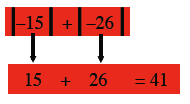Home > MC2 > Chapter 11 > Lesson 11.1.2 > Problem11-19

11-19.
1. Read the Math Notes box for this lesson. Then simplify each of the following expressions. Homework Help ✎

1.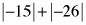2.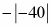3.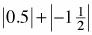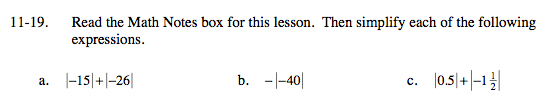Absolute value is the distance a number is from zero on the number line in either direction.
We use the special symbol shown below to indicate the absolute value of any number x.

$|x|$

For example: The absolute value of any number is non-negative.

$|-3|=3\text{ and }|3|=3$